# 精灵的控制

``````auto mySprite = Sprite::create("mysprite.png");
``````## 锚点

``````// DEFAULT anchor point for all Sprites
mySprite->setAnchorPoint(0.5, 0.5);

// bottom left
mySprite->setAnchorPoint(0, 0);

// top left
mySprite->setAnchorPoint(0, 1);

// bottom right
mySprite->setAnchorPoint(1, 0);

// top right
mySprite->setAnchorPoint(1, 1);
``````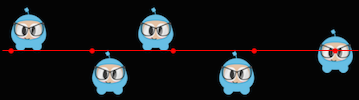## 位置``````// position a sprite to a specific position of x = 100, y = 200.
mySprite->setPosition(Vec2(100, 200));
``````

## 旋转

``````// rotate sprite by +20 degrees
mySprite->setRotation(20.0f);

// rotate sprite by -20 degrees
mySprite->setRotation(-20.0f);

// rotate sprite by +60 degrees
mySprite->setRotation(60.0f);

// rotate sprite by -60 degrees
mySprite->setRotation(-60.0f);
``````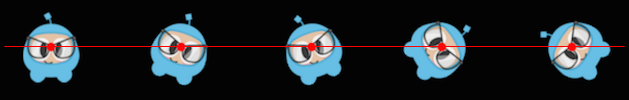## 缩放

``````// increases X and Y size by 2.0 uniformly
mySprite->setScale(2.0);

// increases just X scale by 2.0
mySprite->setScaleX(2.0);

// increases just Y scale by 2.0
mySprite->setScaleY(2.0);
``````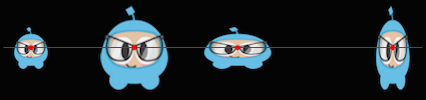## 倾斜

``````// adjusts the X skew by 20.0
mySprite->setSkewX(20.0f);

// adjusts the Y skew by 20.0
mySprite->setSkewY(20.0f);
``````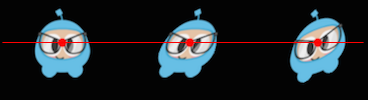## 颜色

``````// set the color by passing in a pre-defined Color3B object.
mySprite->setColor(Color3B::WHITE);

// Set the color by passing in a Color3B object.
mySprite->setColor(Color3B(255, 255, 255)); // Same as Color3B::WHITE
``````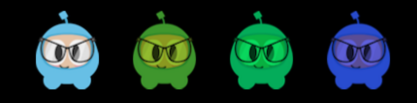## 透明度

``````// Set the opacity to 30, which makes this sprite 11.7% opaque.
// (30 divided by 256 equals 0.1171875...)
mySprite->setOpacity(30);
``````